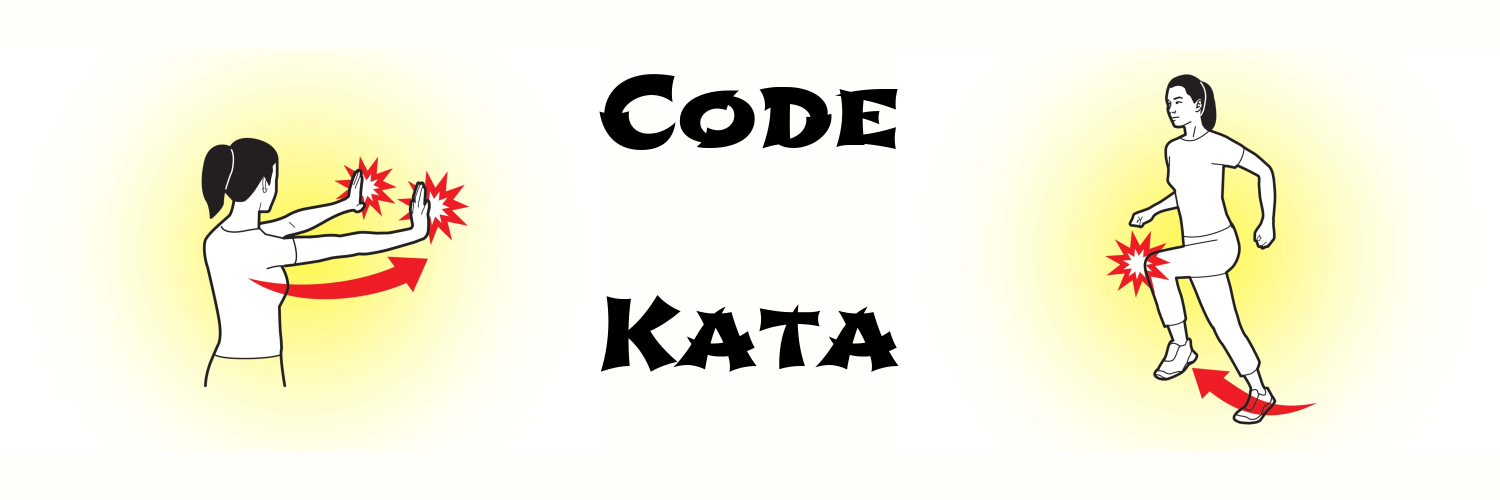# programming kata day 5# Problem

Write a function to determine if a number is a triangular number.

# Solution 1

(defn nth-triangle [n]
(apply + (range (inc n))))

(defn is-triangle-number [n]
(loop [i 0]
(cond (> n (nth-triangle i))
(recur (inc i))
(= n (nth-triangle i))
true
:else
false)))

# Solution 2

(defn is-triangle-number [n]
(loop [tri 0
i 1]
(cond (> n tri)
(recur (+ tri i) (inc i))
(= n tri)
true
:else
false)))

# Note

Solution 1 is the first that came to my mind, but it has a typical problem: redundant calculation. Solution 2 solves it by keeping the last triangular number.